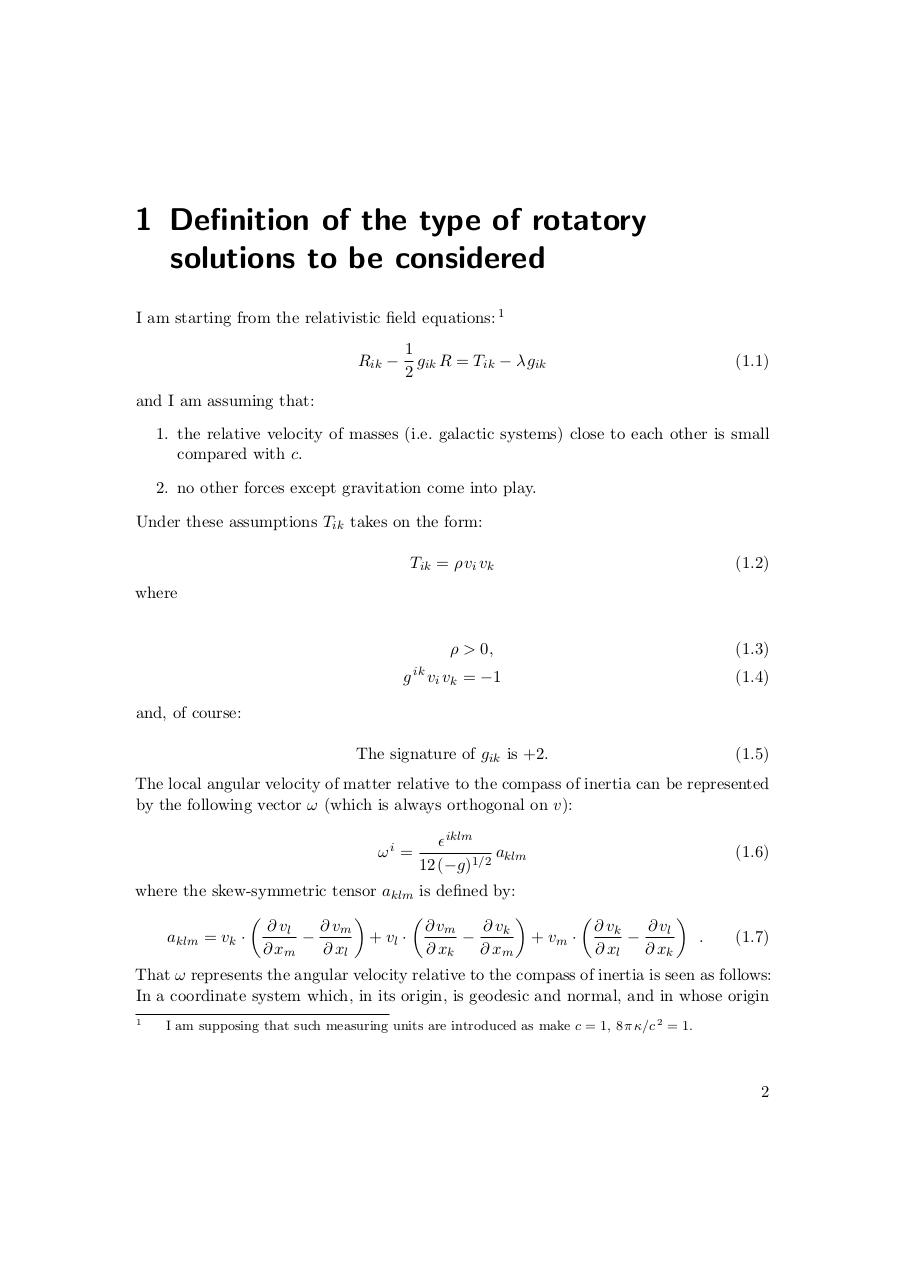# Kurt Gödel. Rotating Universes In General Relativity Theory.pdfPage 1 2 3 4 5 6 7 8 9 10 11

#### Text preview

1 Definition of the type of rotatory
solutions to be considered
I am starting from the relativistic field equations: 1
Rik −

1
gik R = Tik − λ gik
2

(1.1)

and I am assuming that:
1. the relative velocity of masses (i.e. galactic systems) close to each other is small
compared with c.
2. no other forces except gravitation come into play.
Under these assumptions Tik takes on the form:
Tik = ρ vi vk

(1.2)

ρ &gt; 0,

(1.3)

where

ik

g vi vk = −1

(1.4)

The signature of gik is +2.

(1.5)

and, of course:

The local angular velocity of matter relative to the compass of inertia can be represented
by the following vector ω (which is always orthogonal on v):
ωi =

iklm
aklm
12 (−g)1/2

where the skew-symmetric tensor aklm is defined by:

∂ vm
∂ vm
∂ vk
∂ vk
∂ vl
∂ vl

+ vl ·

+ vm ·

.
aklm = vk ·
∂ xm
∂ xl
∂ xk
∂ xm
∂ xl
∂ xk

(1.6)

(1.7)

That ω represents the angular velocity relative to the compass of inertia is seen as follows:
In a coordinate system which, in its origin, is geodesic and normal, and in whose origin
1

I am supposing that such measuring units are introduced as make c = 1, 8 π κ/c 2 = 1.

2